# 球形界面对向列相液晶系统中+1 boojum缺陷结构转变的影响The Effect of Spherical Interface on the Structural Transition of +1 Boojum within the Nematic System

DOI: 10.12677/MS.2018.88105, PDF, HTML, XML, 下载: 629  浏览: 988  国家自然科学基金支持

Abstract: Based on the Landau-de Gennes theory, using a two-dimensional finite-difference iterative method, a cylinder-inscribed-sphere (CIS) nematic liquid crystal (NLC) system with +1 boojum under nanoscale was established. The structural transition of +1 boojum defect induced by the anchoring conditions on the spherical interface was firstly studied. Then, by comparing with the structural transition process of +1 boojum defect in the cylindrical liquid crystal cell, we studied the effect of spherical surface on the structural transition of +1 boojum defect. Results show that, the structure of +1 boojum will shrink and system will not reach the order reconstruction (OR) state. This phe-nomenon is mainly due to the geometry of spherical interface. Furthermore, the result is irrelevant to the anchoring conditions (weak or strong) on the boundary.

1. 引言

Karlj和Rosso等人 运用Landau-de Gennes 理论 ，研究了一个包含+1缺陷的轴对称圆柱形液晶系统，上下基板分别为足够强的沿面锚定和足够强的垂直锚定，这样的几何限制和边界条件会在上基板诱导出一个boojum缺陷。当限制条件为几十纳米时，称这样的缺陷结构为手指形boojum (fingered boojum)。随后，Xiaoyu Jiang等人 同样运用Landau-de Gennes理论和二维有限差分迭代法 研究了一个包含−1缺陷的圆柱形简并混合排列向列相(DHAN)液晶盒，上下基板分别为强的垂直锚定和强的沿面锚定。当盒厚较大时会在下基板中心诱导一个boojum缺陷，对于该−1 boojum缺陷，其指向矢分布不具有轴对称性，但其双轴性分布具有轴对称性。当液晶的厚度减小到足够小(~20 nm)时，这两种液晶系统内均会诱导出有序重构(order reconstruction, OR)现象，即本征值交换。有序重构(本征值交换)现象首次由Schopohl和Sluckin在 $s=±1/2$ 楔形向错中发现 。本征值交换即两个具有正交指向矢的单轴态在两个基板间互相交换，中间不存在本征矢的转动，但本征值随位置连续变化，且存在一系列双轴态连接两个单轴态。随后，向列相液晶薄盒中的缺陷结构 成为研究热点。其中，陈思博等人在研究包含拓扑缺陷的混合向列相液晶薄盒时发现，当液晶系统的厚度减小到约27.4 nm时，系统由缺陷态转变为有序重构态，即发生本征值交换，我们定义此时的盒厚为临界盒厚 。

2. 几何模型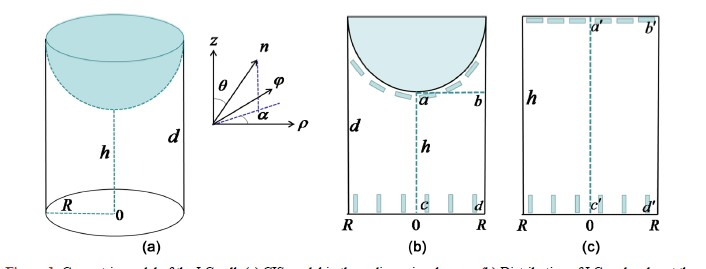Figure 1. Geometric model of the LC cell. (a) CIS model in three-dimensional space; (b) Distribution of LC molecules at the spherical interface and lower substrates in ρ-z plane within CIS model; (c) Distribution of LC molecules at the upper and lower substrates in ρ-z plane within cylinder model

3. 理论方法

$Q=\underset{i=1}{\overset{3}{\sum }}{\lambda }_{i}{e}_{i}\otimes {e}_{i}$ (1)

$Q=S\left(n\otimes n-\frac{1}{3}I\right)$ (2)

${\beta }^{2}=1-\frac{6{\left[tr\left({Q}^{3}\right)\right]}^{2}}{{\left[tr\left({Q}^{2}\right)\right]}^{3}}$ (3)

3.1. 自由能密度

$F\left(Q\right)={\int }_{V}\left({f}_{\text{bulk}}+{f}_{\text{elastic}}\right)\text{d}V+{\int }_{s}{f}_{\text{surface}}\text{d}s$ (4)

${f}_{\text{bulk}}=\frac{A}{2}tr{Q}^{2}-\frac{B}{3}tr{Q}^{3}+\frac{C}{4}{\left(tr{Q}^{2}\right)}^{2}$ (5)

${f}_{\text{elastic}}=\frac{1}{2}{L}_{1}{Q}_{ij,k}{Q}_{ij,k}+\frac{1}{2}{L}_{2}{Q}_{ij,j}{Q}_{ik,k}+\frac{1}{2}{L}_{3}{Q}_{lk}{Q}_{ij,l}{Q}_{ij,k}$ (6)

${f}_{\text{elastic}}=\frac{1}{2}{L}_{1}{Q}_{ij,k}{Q}_{ij,k}$ (7)

3.2. 边界条件

${f}_{s}=\frac{1}{2}{W}_{s}tr{\left(Q-{Q}_{s}\right)}^{2}$ (8)

$\frac{\partial {f}_{s}}{\partial {Q}_{ij}}+\frac{\partial f}{\partial {Q}_{ij,k}}{\stackrel{\to }{v}}_{k}+{\lambda }_{0}{\delta }_{ij}=0$ (9)

3.3. 无量纲化

$\begin{array}{c}{\stackrel{˜}{f}}_{\text{bulk}}=\frac{\stackrel{˜}{A}}{12}tr{\stackrel{˜}{Q}}^{2}-\frac{1}{3}tr{\stackrel{˜}{Q}}^{3}+\frac{1}{16}{\left(tr{\stackrel{˜}{Q}}^{2}\right)}^{2}\\ =\frac{\stackrel{˜}{A}}{12}\left({\stackrel{˜}{Q}}^{2}{}_{\rho \rho }+{\stackrel{˜}{Q}}^{2}{}_{\phi \phi }+{\stackrel{˜}{Q}}^{2}{}_{zz}+2{\stackrel{˜}{Q}}^{2}{}_{\rho \phi }+2{\stackrel{˜}{Q}}^{2}{}_{\rho z}+2{\stackrel{˜}{Q}}^{2}{}_{\phi z}\right)-\frac{1}{3}\left[{\left({\stackrel{˜}{Q}}^{2}\right)}_{\rho \rho }{\stackrel{˜}{Q}}_{\rho \rho }+{\left({\stackrel{˜}{Q}}^{2}\right)}_{\phi \phi }{\stackrel{˜}{Q}}_{\phi \phi }\\ \text{\hspace{0.17em}}\text{\hspace{0.17em}}+{\left({\stackrel{˜}{Q}}^{2}\right)}_{zz}{\stackrel{˜}{Q}}_{zz}+2{\left({\stackrel{˜}{Q}}^{2}\right)}_{\rho \phi }{\stackrel{˜}{Q}}_{\rho \phi }+2{\left({\stackrel{˜}{Q}}^{2}\right)}_{\rho z}{\stackrel{˜}{Q}}_{\rho z}+2{\left({\stackrel{˜}{Q}}^{2}\right)}_{\phi z}{\stackrel{˜}{Q}}_{\phi z}\right]\\ \text{\hspace{0.17em}}\text{\hspace{0.17em}}+\frac{1}{16}{\left({\stackrel{˜}{Q}}^{2}{}_{\rho \rho }+{\stackrel{˜}{Q}}^{2}{}_{\phi \phi }+{\stackrel{˜}{Q}}^{2}{}_{zz}+2{\stackrel{˜}{Q}}^{2}{}_{\rho \phi }+2{\stackrel{˜}{Q}}^{2}{}_{\rho z}+2{\stackrel{˜}{Q}}^{2}{}_{\phi z}\right)}^{2}\end{array}$ (10)

$\begin{array}{c}{\stackrel{˜}{f}}_{\text{elastic}}=\frac{1}{2}{|\stackrel{˜}{\nabla }\stackrel{˜}{Q}|}^{2}\\ =\frac{1}{2}\left[{\stackrel{˜}{Q}}^{2}{}_{\rho \rho ,\stackrel{˜}{\rho }}+{\stackrel{˜}{Q}}^{2}{}_{\rho \rho ,\stackrel{˜}{z}}+{\stackrel{˜}{Q}}^{2}{}_{\phi \phi ,\stackrel{˜}{\rho }}+{\stackrel{˜}{Q}}^{2}{}_{\phi \phi ,\stackrel{˜}{z}}+{\stackrel{˜}{Q}}^{2}{}_{zz,\stackrel{˜}{\rho }}+{\stackrel{˜}{Q}}^{2}{}_{zz,\stackrel{˜}{z}}\begin{array}{c}\\ \end{array}\\ \text{\hspace{0.17em}}\text{\hspace{0.17em}}+2\left({\stackrel{˜}{Q}}^{2}{}_{\rho \phi ,\stackrel{˜}{\rho }}+{\stackrel{˜}{Q}}^{2}{}_{\rho \phi ,\stackrel{˜}{z}}\right)+2\left({\stackrel{˜}{Q}}^{2}{}_{\rho z,\stackrel{˜}{\rho }}+{\stackrel{˜}{Q}}^{2}{}_{\rho z,\stackrel{˜}{z}}\right)+2\left({\stackrel{˜}{Q}}^{2}{}_{\phi z,\stackrel{˜}{\rho }}+{\stackrel{˜}{Q}}^{2}{}_{\phi z,\stackrel{˜}{z}}\right)\\ \text{\hspace{0.17em}}\text{\hspace{0.17em}}+\frac{2}{{\stackrel{˜}{\rho }}^{2}}\left({\stackrel{˜}{Q}}^{2}{}_{\rho \rho }+{\stackrel{˜}{Q}}^{2}{}_{\phi \phi }+4{\stackrel{˜}{Q}}^{2}{}_{\rho \phi }+{\stackrel{˜}{Q}}^{2}{}_{\rho z}+{\stackrel{˜}{Q}}^{2}{}_{\phi z}-2{\stackrel{˜}{Q}}_{\rho \rho }{\stackrel{˜}{Q}}_{\phi \phi }\right)\right]\end{array}$ (11)

$\begin{array}{c}{\stackrel{˜}{f}}_{s}=\frac{1}{2}{\stackrel{˜}{W}}_{s}\xi tr{\left(\stackrel{˜}{Q}-{\stackrel{˜}{Q}}_{s}\right)}^{2}\\ =\frac{1}{2}{\stackrel{˜}{W}}_{s}\xi \left[{\stackrel{˜}{Q}}^{2}{}_{\rho \rho }+{\stackrel{˜}{Q}}^{2}{}_{\phi \phi }+{\stackrel{˜}{Q}}^{2}{}_{zz}+2{\stackrel{˜}{Q}}^{2}{}_{\rho \phi }+2{\stackrel{˜}{Q}}^{2}{}_{\rho z}+2{\stackrel{˜}{Q}}^{2}{}_{\phi z}\\ \text{\hspace{0.17em}}\text{\hspace{0.17em}}+{\stackrel{˜}{Q}}^{2}{}_{s\rho \rho }+{\stackrel{˜}{Q}}^{2}{}_{s\phi \phi }+{\stackrel{˜}{Q}}^{2}{}_{szz}+2{\stackrel{˜}{Q}}^{2}{}_{s\rho \phi }+2{\stackrel{˜}{Q}}^{2}{}_{s\rho z}+2{\stackrel{˜}{Q}}^{2}{}_{s\phi z}\\ \text{\hspace{0.17em}}\text{\hspace{0.17em}}-2\left({\stackrel{˜}{Q}}^{2}{}_{\rho \rho }{\stackrel{˜}{Q}}^{2}{}_{s\rho \rho }+{\stackrel{˜}{Q}}^{2}{}_{\phi \phi }{\stackrel{˜}{Q}}^{2}{}_{s\phi \phi }+{\stackrel{˜}{Q}}^{2}{}_{zz}{\stackrel{˜}{Q}}^{2}{}_{szz}+2{\stackrel{˜}{Q}}^{2}{}_{\rho \phi }{\stackrel{˜}{Q}}^{2}{}_{s\rho \phi }+2{\stackrel{˜}{Q}}^{2}{}_{\rho z}{\stackrel{˜}{Q}}^{2}{}_{s\rho z}+2{\stackrel{˜}{Q}}^{2}{}_{\phi z}{\stackrel{˜}{Q}}^{2}{}_{s\phi z}\right)\right]\end{array}$ (12)

$\frac{\partial {\stackrel{˜}{f}}_{s}}{\partial {\stackrel{˜}{Q}}_{ij}}+\xi \frac{\partial \stackrel{˜}{f}}{\partial {\stackrel{˜}{Q}}_{ij,\stackrel{˜}{k}}}{v}_{k}+{\lambda }_{0}{\delta }_{ij}=0$ (13)

3.4. 动力学求解

$\frac{\partial Q}{\partial t}=\Gamma \left[-\frac{\delta f}{\delta Q}+\frac{1}{3}tr\left(\frac{\delta f}{\delta Q}\right)I\right]$ (14)

$\frac{\partial \stackrel{˜}{Q}}{\partial t}=\stackrel{˜}{\Gamma }\left[-\frac{\delta \stackrel{˜}{f}}{\delta \stackrel{˜}{Q}}+\frac{1}{3}tr\left(\frac{\delta \stackrel{˜}{f}}{\delta \stackrel{˜}{Q}}\right)I\right]$ (15)

$\left[\stackrel{˜}{Q}\left(t+\Delta t\right)-\stackrel{˜}{Q}\left(t\right)\right]/\Delta t=-\stackrel{˜}{\Gamma }\left[-\frac{\delta \stackrel{˜}{f}}{\delta \stackrel{˜}{Q}}+\frac{1}{3}tr\left(\frac{\delta \stackrel{˜}{f}}{\delta \stackrel{˜}{Q}}\right)I\right]$ (16)

$\frac{\delta \stackrel{˜}{f}}{\delta \stackrel{˜}{Q}}=\frac{\delta \stackrel{˜}{f}}{\delta {\stackrel{˜}{Q}}_{ij}}-\frac{\partial }{\partial \stackrel{˜}{\rho }}\frac{\delta \stackrel{˜}{f}}{\delta {\stackrel{˜}{Q}}_{ij,\stackrel{˜}{\rho }}}-\frac{1}{\stackrel{˜}{\rho }}\frac{\delta \stackrel{˜}{f}}{\delta {\stackrel{˜}{Q}}_{ij,\stackrel{˜}{\rho }}}-\frac{\partial }{\partial \stackrel{˜}{z}}\frac{\delta \stackrel{˜}{f}}{\delta {\stackrel{˜}{Q}}_{ij,\stackrel{˜}{z}}}$ (17)

4. 数值结果

4.1. 强锚定边界条件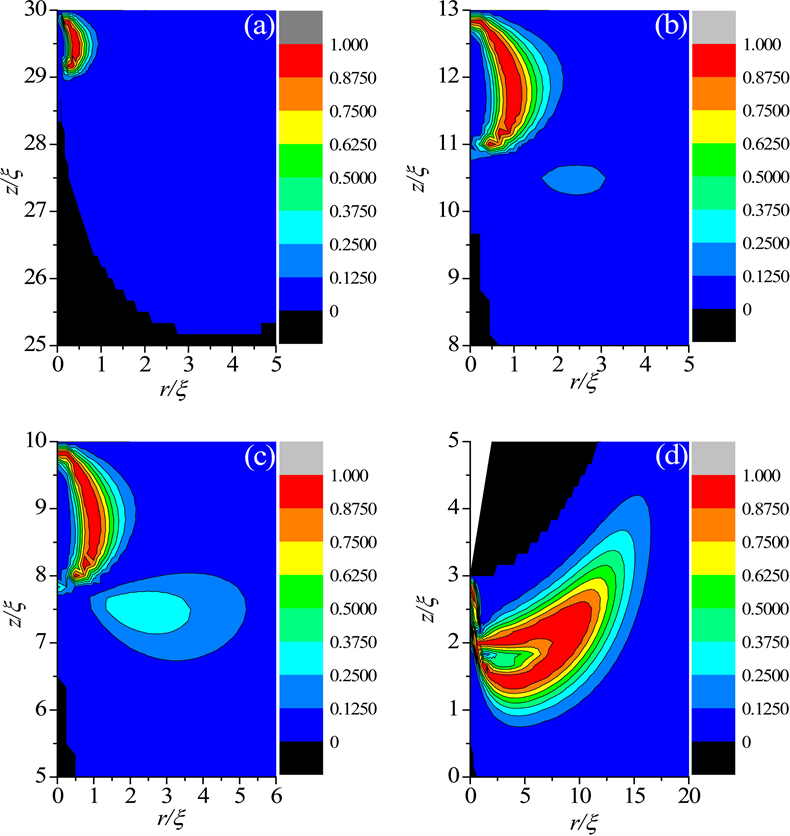Figure 2. Biaxiality profiles of partial enlargement of +1 boojum defect in CIS LC cell. (a) $r=10\xi ,h=30\xi$ ; (b) $r=27\xi ,h=13\xi$ ; (c) $r=30\xi ,h=10\xi$ ; (d) $r=37\xi ,h=3\xi$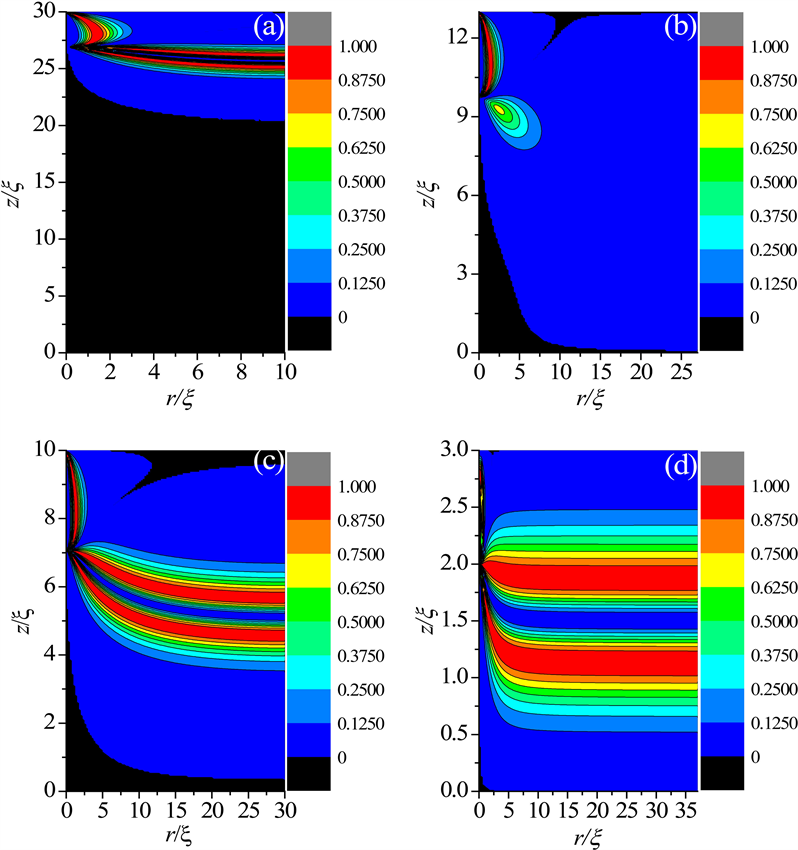Figure 3. Biaxiality profiles about the structural transition of the +1 boojum in the cylindrical LC cell with the increases of radius and decreases of the cell gap. (a) $r=10\xi ,h=30\xi$ ; (b) $r=27\xi ,h=13\xi$ ; (c) $r=30\xi ,h=10\xi$ ; (d) $r=37\xi ,h=3\xi$Figure 4. Profiles about the eigenvalue configurations of the order parameter tensor Q at the symmetry axis $r=0$ when $r=27\xi ,h=13\xi$ . (a) Eigenvalue profiles within the CIS LC cell; (b) Eigenvalue profiles within the cylindrical LC cell. (c) Comparison of the positions of the fingertips in the +1 boojum defect structure in the LC cells of the two models with different cell gaps

4.2. 弱锚定边界条件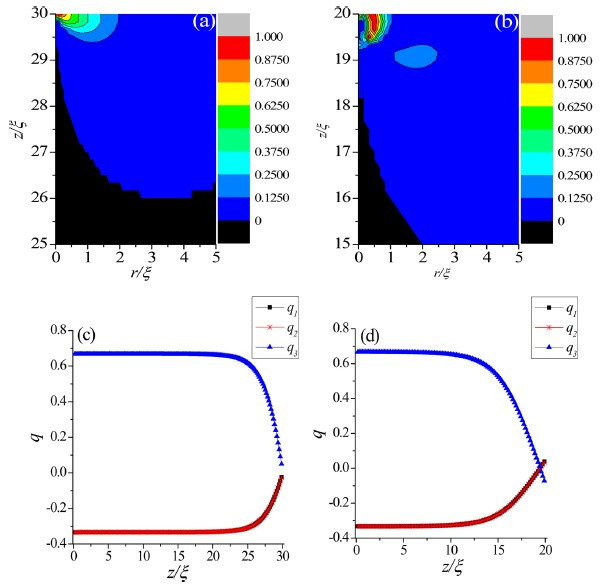Figure 5. Profiles of biaxiality partial enlargement of the boojum defect in the CIS LC cell and the eigenvalue diagrams of the order parameter tensor Q at the symmetry axis $r=0$ under the weak anchoring boundary condition when $W=1×{10}^{-3}\text{J}/{\text{m}}^{\text{2}}$ . (a) and (c) $r=10\xi ,h=30\xi$ ；(b) and (d) $r=20\xi ,h=20\xi$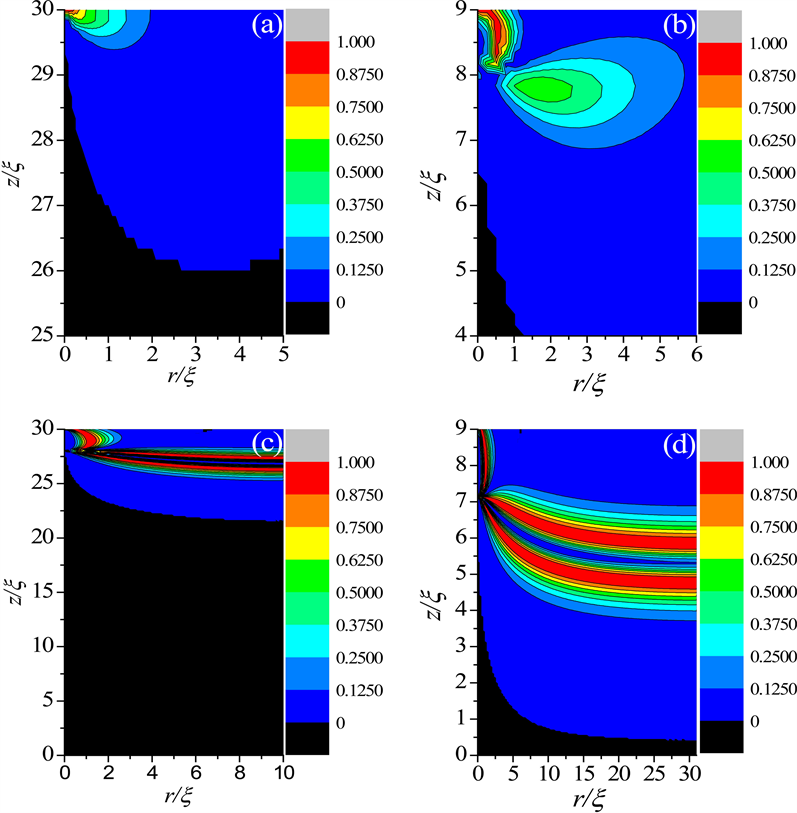Figure 6. Biaxiality enlargement profiles under the weak anchoring boundary condition when $W=1×{10}^{-3}\text{J}/{\text{m}}^{\text{2}}$ in two models. (a) and (b) are partial enlarged details of the biaxiality configurations in the CIS LC cell; (c) and (d) are biaxiality configurations in the cylindrical LC cell. In (a) and (c), $r=10\xi ,h=30\xi$ ; (b) and (d), $r=31\xi ,h=9\xi$

5. 结论

Boojum缺陷在液晶的非显示方面具有重要的应用。例如，利用圆环状胶体在向列相液晶中产生的boojum缺陷实现了新型的二维材料 ；通过球形胶体在向列相液晶中产生的boojum缺陷捕获了等离子体纳米粒子，并形成新的二聚体单元 等。

NOTES

*通讯作者。

  Points, K.M. (1983) Lines and Walls: in Liquid Crystals, Magnetic Systems and Various Disordered Media. Wiley, New York.  Volovik, G.E. and Lavrentovich, O.D. (1983) Topological Dynamics of Defects: Boojums in Nematic Drops. Zh Eksp Teor Fiz, 85, 1159-1166.  Volovik, G.E. (1978) Topological Singularities on the Surface of an Ordered System. Pis’ma Zh Eksp Teor Fiz, 28, 59-61.  Candua, S., Le, R.P. and Debeauvais, F. (1973) Magnetic Field Effects in Nematic and Cholesteric Droplets Suspended in an Isotropic Liquid. Molecular Crystals and Liquid Crystals, 23, 283-297. https://doi.org/10.1080/15421407308083378  Kurik, M.V. and Lavrentovich, O.D. (1982) Negative-Positive Monopole Transition in Cholesteric Liquid Crystal. Pis’ma Zh Eksp Teor Fiz, 35, 444-447.  Kralj, S., Rosso, R. and Virga, E.G. (2010) Finite-Size Effects on Order Reconstruction around Nematic Defects. Physical Review E, 81, 1-15. https://doi.org/10.1103/PhysRevE.81.021702  Kralj, S., Rosso, R. and Virga, E.G. (2008) Fingered Core Structure of Nematic Boojums. Physical Review E, 78, 1-4. https://doi.org/10.1103/PhysRevE.78.031701  De Genns, P.G. and Prost, J. (1993) The Physics of Liquid Crystals. Oxford University Press, Oxford.  Jiang, X.Y., Zhou, X. and Zhang, Z.D. (2016) Landau-de Gennes Theory for a -1 Boojum in a Degenerate Hybrid-Aligned Nematic Cell. Liquid Crystals, 69, 490-499.  Zhou, X. and Zhang, Z.D. (2014) Dynamics of Order Reconstruction in Nanoconfined Twisted Nematic Cells with a Topological Defect. Liquid Crystals, 41, 1219-1228. https://doi.org/10.1080/02678292.2014.912689  Schopohl, N. and Sluckin, T.J. (1987) Defect Core Structure in Nematic Liquid Crystals. Physical Review Letters, 59, 2582-2584. https://doi.org/10.1103/PhysRevLett.59.2582  Bisi, F., Virga, E.G. and Durand, G.E. (2004) Nanomechanics of Order Reconstruction in Nematic Liquid Crystals. Physical Review E, 70, 174-195. https://doi.org/10.1103/PhysRevE.70.042701  Carbone, G., Lombardo, G., Barberi, R., et al. (2009) Me-chanically Induced Biaxial Transition in a Nanoconfined Nematic Liquid Crystal with a Topological Defect. Physical Review Letters, 103, Article ID: 167801.  Wang, Y.C., Zhou, X., Ye, W.J. and Zhang, Z.D. (2015) New Surface Order Reconstruction Induced by Electric Field Application in a Nanoconfined HAN Cell with a Topological Defect. AIP Advances, 5, 1-10. https://doi.org/10.1063/1.4934672  陈思博, 王永超, 周璇, 等. 电场诱导纳米结构HAN液晶盒中新型有序重构过程的描述[J]. 液晶与显示, 2016, 31(9): 853-861.  Tasinkevych, M., Silvestre, N.M. and Telo da Gama, M.M. (2012) Liquid Crystal Boojum-Colloids. New Journal of Physics, 14, Article ID: 073030. https://doi.org/10.1088/1367-2630/14/7/073030  Lubensky, T.C., Pettey, D., Currier, N. and Stark, H. (1998) Topological Defects and Interactions in Nematic Emulsions. Physical Review E, 57, 610. https://doi.org/10.1103/PhysRevE.57.610  Mermin, N.D. (1997) Games to Play with 3He-A. Physica B + C, 90, 1-10. https://doi.org/10.1016/0378-4363(77)90003-1  Mermin, N.D. (1990) Boojum All the Way through. Cam-bridge University Press, Cambridge. https://doi.org/10.1017/CBO9780511608216  Poulin, P. and Weitz, D.A. (1998) Inverted and Multiple Ne-matic Emulsions. Physical Review E, 57, 626-637. https://doi.org/10.1103/PhysRevE.57.626  Virga, E.G. (1994) Variational Theories for Liquid Crystals. Chapman & Hall, London. https://doi.org/10.1007/978-1-4899-2867-2  Kaiser, P., Wiese, W. and Hess, S. (1992) Stability and Instability of a Uniaxial Alignment against Biaxial Distortions in the Isotropic and Nematic Phases of Liquid Crystals. Journal of Non-Equilibrium Thermodynamics, 17, 153-169. https://doi.org/10.1515/jnet.1992.17.2.153  Kleman, M. and Lavrentovich, O.D. (2002) Soft Matter Physics. Berlin, Springer.  Amoddeo, A., Barberi, R. and Lombardo, G. (2011) Electric Field-Induced Fast Nematic Order Dynamics. Liquid Crystals, 38, 93-103. https://doi.org/10.1080/02678292.2010.530298  Nolibi, M. and Du-rand, G. (1992) Disorientation Induced Disordering at a Nematic Liquid Crystal Solid Interface. Physical Review A, 46, 174-177.  Lombardo, G., Ayeb, H. and Barberi, R. (2008) Dynamical Numerical Model for Nematic Order Re-construction. Physical Review E, 77, 1-10. https://doi.org/10.1103/PhysRevE.77.051708  Guzmán, O., Abbott, N.L. and De Pablo, J.J. (2005) Quenched Disorder in a Liquid-Crystal Biosensor: Adsorbed Nanoparticles at Confining Walls. The Journal of Chemical Physics, 122, 1-10. https://doi.org/10.1063/1.1896354  Qian, T.-Z. and Sheng, P. (1997) Orientational States and Phase Transitions Induced by Microtextured Substrates. Physical Review E, 55, 7111-7120. https://doi.org/10.1103/PhysRevE.55.7111  Tian, Y. and Zhang, Z.D. (2015) Interaction between a + 1 Defect in a Nematic Thin Film and the Surface Wall and Flxoelectric Response at the Defect Site. Liquid Crystals, 42, 288-297. https://doi.org/10.1080/02678292.2014.984647  Liu, Q., Bohdan, S., Mykola, T. and Smalyukh, I.I. (2013) Nematic Liquid Crystal Boojums with Handles on Colloidal Handlebodies. PNAS, 110, 9231-9236. https://doi.org/10.1073/pnas.1301464110  Senyuk, B., Evans, J.S., Ackenman, P.J., et al. (2012) Shape-Dependent Oriented Trapping and Scaffolding of Plasmonic Nanoparticles by Topological Defects for Self-Assembly of Colloidal Dimers in Liquid Crystals. Nano Letters, 12, 955-963. https://doi.org/10.1021/nl204030t